# AP Physics B : Understanding Resistors and Resistance

## Example Questions

### Example Question #3 : Electric Circuits

Which of the following changes to a copper wire will lead to the greatest decrease in voltage?

Increasing the cross-sectional area of the copper wire by a factor of two

Increasing the current through the copper wire by a factor of two

Increasing the length of the copper wire by a factor of two

Replacing the copper wire with a more conductive material

Increasing the cross-sectional area of the copper wire by a factor of two

Explanation:

According to Ohm’s law a decrease in current and/or resistance will lead to a decrease in voltage, since voltage is directly proportional to both current and resistance.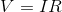Increasing the current will not decrease voltage. Remember that resistance is defined as: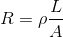In this formula,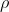is the resistivity,is the length of the wire, andis the cross-sectional area of the wire. Increasing length will lead to an increase in resistance and voltage; however, increasing the area will lead to a decrease in resistance and, subsequently, a decrease in voltage.

The only answer that will lead to a decrease in voltage is the choice to increase the cross-sectional area of the wire.

Replacing the copper wire with a more conductive material will increase the resistivity, which will subsequently increase resistance and voltage.

### Example Question #4 : Electric Circuits

A student assembles a circuit made up of a voltage source and two resistors. All three circuit elements are connected in parallel. The voltage across the voltage source is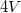and the resistance of the resistors are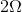and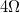respectively. Which of the following is true of this circuit?

The voltage across the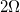resistor will equal the voltage across theresistor

The current through theresistor will equal the current through theresistor

The current through theresistor will be less than the current through theresistor

The voltage across theresistor will be less than the voltage across theresistor

The voltage across theresistor will equal the voltage across theresistor
When circuit elements, such as resistors, are connected in parallel they will have the same voltage drop. The current through theresistor will be greater than current through theresistor, but their voltages will be equal. According to Ohm’s law the current flowing through the smaller resistor will be larger. Ohm’s law can be rearranged to solve for current as follows: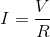Since voltage is the same across resistors, the lower resistance () will have more current flowing through it since current is inversely proportional to resistance.